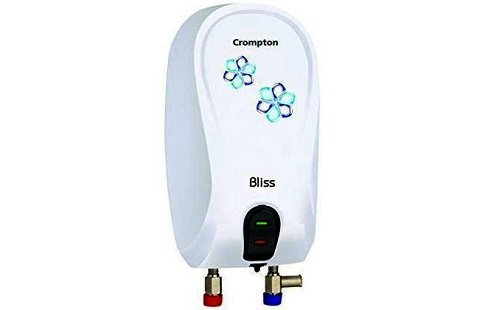# 3-Litre Geyser Power Consumption Details – A Deep Analyses

Water geysers are now an important part of everyone’s kitchen. Geysers provide us with hot water whenever you need it. Now the demand for geysers is at the top. Geysers help us in many ways, but it is most important to consider that how much electricity it consumes while working. Always buy a geyser that does not consume a large amount of energy, it will make a high electric bill.

Geysers are available from 3 to 25-litre capacity. Every geyser uses energy based on many watts it consumes in one hour. Geysers heat the cold water by the heat induction through the coil. These coils get hot when connected to the electricity. These coils are available in different heating capacities. Big geysers have a large coil in them and the consumed electricity depends on the coils.If the coil is 1000 W or 1 KW, then it will consume 1 kWh or 1 unit in 1 hour. This is one of the simplest calculations to understand the consumption of the power by the geysers. You can very easily calculate the energy consumed by the geyser by understanding this.

3-litre geysers can have 3000 W to 4500 W coil. More the watts consumed an hour, less the time is consumed for the heating water. Thus, if you want a storage geyser with power efficiency, buy a geyser that consumes fewer watts. But, if you don’t care about the energy consumed, then always buy the 4500 watts 3-litre geyser. Because it will take a couple of seconds to heat the water and saves your time.

Most of the three-litre geysers are provided in the two-energy range, the first is 3 KW, and the second is 4.5 KW. Both are best for household uses.

Calculation Method

To easily understand the power consumption by the 3-litre geyser, read the following parameters.

• Wattage

Wattage is the main parameter of any electronic device, which provides information about energy consumption through the device. Watts is the unit of electric power, if something is of 1500 W, it means that it consumes 1500 Watt in one hour. If you calculate the power required by the geyser for heating, you can calculate the accurate consumption.

• Operational hours

This is the amount of time in which the appliance is continuously working. This time is important while calculating the energy consumption by the 3-litre geyser. Without the time factor, you can’t calculate the energy consumed by the geyser. However, there is one more factor that matters during the calculation time and it is the auto cut feature. Many geysers come with auto cut functionality to reduce electricity wastage.

• Price of electricity

After calculating all the parameters, you can easily calculate the units of energy consumed by the geyser. By multiplying it by the price of one unit you can easily calculate the bill. On the other hand, the star rating on the electric geyser explains the power efficiency. This rating might not seem important, but if you want fewer electricity bills, choose a geyser with a higher star rating.